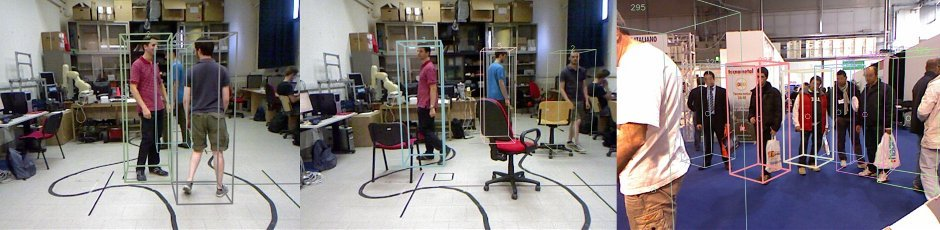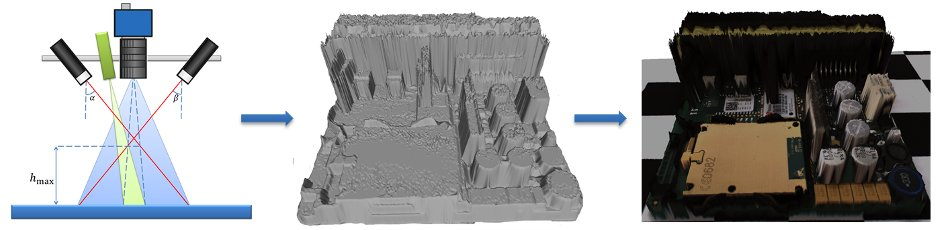•••••﻿

Given two obstacles, make the robot go around the first one and stops 3 cm behind the second one for 10 seconds and finally come back, as in Figure 1.## At the end of the experience...

### Objectives

• Make the robot reach the second obstacle
• Go back to the starting position
• Understand the basic ROS concepts (launcher, compiler and publisher/subscriber)
• Use the gyroscope to improve the robot performances

### Plus

• Use the thrid engine to move the ultrasonic sensor
• Object Oriented (OO) approach

### Challenges

• Unknow distance between the two obstacles

### What we want

• Code (BitBucket)
• Report (PDF using BitBucket) containing
• The algorithm overview
• The procedures to make the robot go straight and turn
• What are the main robot problems and why
• The Launcher description
• The CMakeLists.txt description

## Explanation

### Preliminary steps

cd ~/Workspace/ros/catkin_ws/src
cd .. 
catkin_make --force-cmake -G"Eclipse CDT4 - Unix Makefiles"

roslaunch nxt_unipd nxt_lab.launch
roslaunch nxt_unipd teleop_keyboard.launch


### Robot configuration

nxt_lab.yaml

 -  type: ultrasonic
name: ultrasonic_sensor
port: PORT_4
min_range: 0.01
max_range: 2.5
desired_frequency: 5.0


### Program launcher

nxt_lab.launch

  <group ns="base_parameters">
<param name="r_wheel_joint" value="r_wheel_joint"/>
<param name="l_wheel_joint" value="l_wheel_joint"/>
<param name="wheel_basis" value="0.055"/>
<param name="vel_to_eff" value="0.2"/>
<param name="l_vel_to_eff" value="0.1"/>
<param name="r_vel_to_eff" value="0.1"/>
</group>


### Robot controller

$v_{trans}^{est} \left[i+1\right] = \frac{1}{2} \left( v_{trans}^{est}\left[i\right] + \frac{1}{2} \left( v_{rot}^{reg}\left[i,j_{wheel}^{l} \right] + v_{rot}^{reg}\left[i,j_{wheel}^{r} \right] \right) r_{wheel} \right)$

$v_{rot}^{est} \left[i+1\right] = \frac{1}{2} \left( v_{rot}^{est}\left[i\right] + \frac{1}{2} \left( v_{rot}^{reg}\left[i,j_{wheel}^{l} \right] - v_{rot}^{reg}\left[i,j_{wheel}^{r} \right] \right) \frac{r_{wheel}}{b_{wheel}} \right)$

where:

$$v_{trans}^{est} \left[i\right]$$ is the estimated translational velocity at the instant $$i$$

$$v_{rot}^{est} \left[i\right]$$ is the estimated rotational velocity at the instant $$i$$

$$v_{rot}^{reg}\left[i,j_{wheel}^{l} \right]$$ is the registered rotational velocity for the joint of the left wheel at the instant $$i$$

$$v_{rot}^{reg}\left[i,j_{wheel}^{r} \right]$$ is the registered rotational velocity for the joint of the right wheel at the instant $$i$$

$$r_{wheel}$$ is the wheel radius

$$b_{wheel}$$ is the wheel basis

$v_{trans}^{cmd} \left[i+1\right] = v_{trans}^{des}\left[i\right] + k_{trans} \left( v_{trans}^{des}\left[i \right] - v_{trans}^{est}\left[i +1 \right] \right)$

$v_{rot}^{cmd} \left[i+1\right] = v_{rot}^{des}\left[i\right] + k_{rot} \left( v_{rot}^{des}\left[i \right] - v_{rot}^{est}\left[i \right] \right)$

where:

$$v_{trans}^{cmd} \left[i\right]$$ is the translational velocity applied to the joint  at the instant $$i$$

$$v_{rot}^{cmd} \left[i\right]$$ is the rotational velocity applied to the joint at the instant $$i$$

$$v_{trans}^{des}\left[i \right]$$ is the desired transational velocity for the joint at the instant $$i$$

$$v_{rot}^{des}\left[i \right]$$ is the desired rotational velocity for the joint at the instant $$i$$

$$k_{trans}$$ is the trasational constant

$$k_{rot}$$ is the rotational constant

$F \left[i+1\right] = k_v^F \left( v_{trans}^{cmd}\left[i + 1\right] \frac{1}{r_{wheel}} - v_{rot}^{cmd}\left[i +1 \right] \frac{b_wheel}{r_{wheel}} \right)$

where:

$$F \left[i\right]$$ is the effort applied to the joint at the instant $$i$$

$$k_v^F$$ is the constant to transform velocity to effort

### Robot teleoperation

nxt_key.cpp

    switch(c)
{
case KEYCODE_L:
ROS_DEBUG("LEFT");
angular_ = 2.0;
break;
case KEYCODE_R:
ROS_DEBUG("RIGHT");
angular_ = -2.0;
break;
case KEYCODE_U:
ROS_DEBUG("UP");
linear_ = 0.15;
break;
case KEYCODE_D:
ROS_DEBUG("DOWN");
linear_ = -0.15;
break;
}


teleop_keyboard.launch

<node pkg="nxt_unipd" type="nxt_teleop_key" name="nxt_teleop_key"  output="screen">
<param name="scale_linear" value="1" type="double"/>
<param name="scale_angular" value="1" type="double"/>
</node>


### Sensors messages

nxt_msgs/Range Message

Header header
float64 range
float64 range_min
float64 range_max